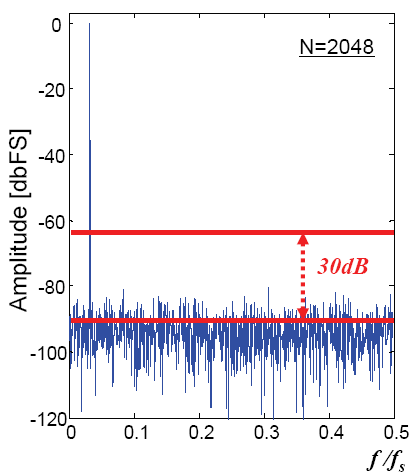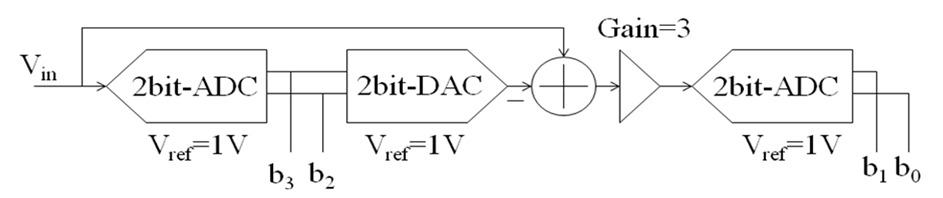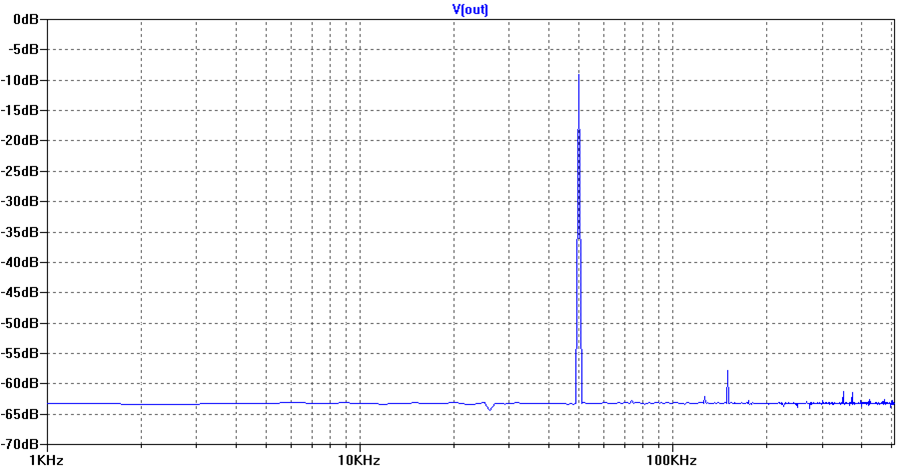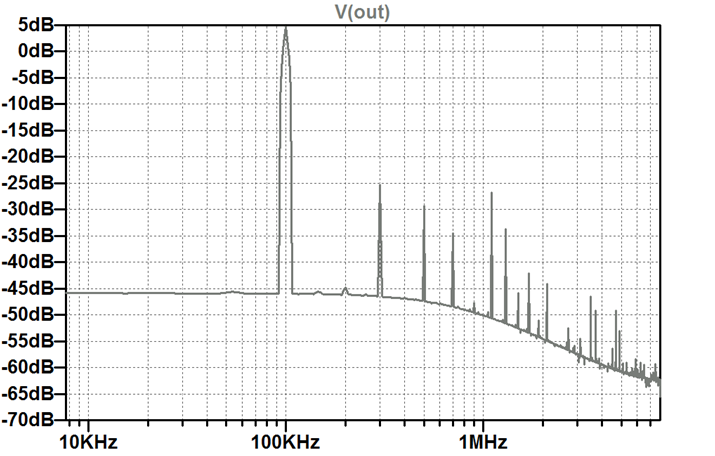# Problems WS2011

## Problem 1: DAC Linearity

For a DAC with VFS= 0.8 V the analog output values were measured according to table 1.
 Digital Code 0 1 10 11 100 101 110 111 Output Voltage [V] 0.03 0.1 0.226 0.31 0.42 0.568 0.65 0.773
Table 1: DAC transfer table

1. Calculate the gain and offset error.
2. Calculate the INL and DNL.
3. What is the Effective number of bits?
4. What is the signal to noise ratio?

## Problem 2: Blocks

List the blocks of an AD converter. What is the task of each block?

## Problem 3: Circuit Analysis

What kind of circuit is shown in the figure?
Explain the operation of the circuit.
How many bits are used by the data converter?
What is the smallest step size of the data converter?
What is the output voltage for the input combination of
MSB..LSB
0101
1001

## Problem 4: Temperature Measurement

A cooking thermometer measures temperature between -20°C and 300°C with +-1°C accuracy.
How many bits should an AD converter have for this application.
What kind of AD converter would you use for this application and why?

## Problem 5: Latency and Bandwith

An 8-bit single slope AD-converter with LSB = 4 mV uses an internal ramp of 2 V/ms.
Calculate the maximum allowed input frequency? (3 points)
Calculate the internal counter frequency? (2 points)
How long does it take to convert 0.38 V? (2 points)
What is the binary output for 0.47 V input voltage? (2 points)

## Problem 6: Metrics

How can you specify DA converters?

## Problem 7: Quantization Noise

Results of a spectral test of a 10bit AD converter are shown in the graph. Explain the graph. Calculate the Signal-to-Quantization-Noise Ratio for a 10bit AD converter. Why is the measure noise floor below the SQNR?

## Problem 8: Test

A ramp test is applied to a 10 bit AD converter with VFS = 10V. The sampling rate fs = 20kHz. The resolution of the test should be 0.4 mV. How long is the test time?# Exam WS2010

The transfer curve of an AD converter results in the following table.
What is the offset error and the LSB value of the ADC (2 points)?
Calculate the INL and DNL values (20 points):
 Input Transition Voltage [V] Digital code 0.090 0001 0.186 0010 0.226 0011 0.322 0100 0.442 0101 0.510 0110 0.562 0111 0.674 1000 0.698 1001 0.798 1010 0.890 1011 0.994 1100 1.018 1101 1.146 1110 1.210 1111
What is the signal to quantization noise ratio (2 points):

## Problem 2:

List the blocks of a digital to analog converter and explain the functions? (8 points)
Calculate the output voltage for a 12-bit DA converter with a minimum step size LSB of 0.2mV for a binary input of (4 points)
010011000011:
010101010010:

## Problem 3:What kind of circuit is shown (2 points)?
Calculate the digital output b3..b0 for 0.9V and 0.3V input voltage. (8 points)
How many output bits and effective bits has the data converter?(2 points)
What is the resolution of the data converter? (2 points)

## Problem 4: Practical Example

You want to build a weight scale. The maximum weight should be 2kg and the resolution should be 0.1g. The output voltage of the sensor at 2kg is 50mV.
1. What is the minimum voltage difference the AD converter has to detect? (2 points)
2. How many bits do you need? (2 points)
3. What is the signal to quantization noise ratio? (2 points)
4. What is the output voltage when you weigh 1438g? (2 points)

## Problem 5: Spectral test and FFT

Analyze the following output spectrum of a Nyquist type DA converter.What is the minimum and maximum frequency in the plot? (1 point)
What frequency has the test signal? (1 point)
What is the sampling frequency? (1 point)
How many points are used for the fft? (1 point)
How many bits has the DA converter? (4 point)

## Problem 6: Latency and Bandwith

An 8-bit single slope AD-converter with LSB=5mV uses an internal ramp of 1V/ms.
Calculate the maximum allowed input frequency? (3 points)
Calculate the internal counter frequency? (2 points)
How long does it take to convert 0.38V? (2 points)
What is the binary output for 0.47V input voltage? (2 points)

## Problem 7:

A 14-Bit ADC is tested using a ramp signal. The LSB is 8mV. The test resolution should be 0.001LSB. The sampling frequency is 800kHz.
1. What is the maximum output voltage? (2 points)
2. How long is the test time? (2 points)
3. What is the ramp slope? (2 points)
4. What kind of AD architecture can be ideally tested with this setup? (4 points)

## Problem 8:

A track and hold circuit of a Nyquist rate 12-bit AD converter with VFS=0.5V should be designed.
1. Why do you need a track and hold circuit? (2 points)
2. What is the difference between a track and hold and sample and hold circuit? (2 points)
3. What is the minimum value for the sampling capacitor C at T=293K? (2 points) (kb= 1.38?10-23 J/K)
4. What is the maximum Resistance allowed to enable a sampling frequency of 50MHz? (2 points)

# Exam WS2011

## Problem 1

A histogramm test of an AD converter gives the following result.
 # of occurences 9 12 15 17 16 13 17 9 Output code 0 1 2 3 4 5 6 7 DNL [LSB] INL [LSB]
How many bits has the AD converter (1 point)?
Calculate the DNL and INL values (9 points)?
What is the resolution of the histogram test? (2 points):
The AD converter has a sampling rate of 100kHz. Calculate the test time for the histogram test. (2 points)
What is the signal to noise ratio and the effective number of bits, if you average 2 output values of the AD converter. (2 points)

## Problem 2

2.1 List and explain errors for DA converters? (10 points)
2.2 A 14-bit DA converter has an offset of 617mV and a measured Vmax=17V. Calculate the output voltage for the following inputs (6 points)
01001100100110:
11010101010010:

## Problem 3

What kind of circuit is shown (2 points)?
The circuit with R = 1kΩ ,C = 20nF is operated with VDD = 1V, VCM = 0.5V and fS = 1 MHz.
A simple decimation filter gives an output of 8 bits.
What is the maximum allowed input voltage? (2 points)
What is the cut-off frequency of the RC input low pass? (1 point)
What is the maximum allowed signal frequency if you want to have an 8-bit value at the output?
Calculate vout(t) for 4µs if Vinin=0.3V. The starting value for vint=VCM and Vout=0V. Assume a constant current through R for a sampling cycle. (8 points)
 t [µs] 0 1 2 3 4 Vin 0.3 V 0.3 V 0.3 V 0.3 V 0.3 V Vint 0.5 V Vout 0 V

## Problem 4: Resistor string DA converter with mismatch

 A real 3-Bit resistor string DA converter with Vref= 4V has some error due to R mismatch. The switches are closed when the control signal is '1'. b signals are the inverted signals. 1. What is the maximum output voltage and the average step size? (3 points) 2. What would be the settling time if all resistors would be 1k?? (2 points) 3. Fill the table for the transfer function, the INL and DNL ? (6 points)

 Code 000 001 010 011 100 101 110 111 Vout[V] INL [LSB] DNL [LSB]

## Problem 5: FFT

 Analyze the following output spectrum of a Nyquist type DA converter. What is the minimum and maximum frequency in the plot? (1 point) What signal level and frequency has the test signal? (2 points) Why does the noise level decrease above 600kHz? (1 point) What is the sampling frequency? (1 point) How many points are used for the fft? (1 point) How many bits has the DA converter? (4 point) How many periods of the test signal are measured? (1 point) What is the spurious free dynamic range? (1 point)## Problem 6: Latency and Bandwith

A digital voltmeter can display positive and negative values with 5 digits. It uses a dual-slope converter running at clock speed of 100MHz with 10V reference voltage.

How many bits are used for the AD converter? (3 points)
What is the smallest voltage, which can be detected? (1 point)
Calculate the maximum allowed input frequency? (3 points)
How long does it take to convert 1.57V? (2 points)
What is the internal binary output for 0.8450V input voltage? (2 points)

## Problem 7

A 10-Bit ADC is tested using a ramp signal. The LSB is 8mV. The test resolution should be 0.005LSB. The sampling frequency is 200kHz.
1. What is the maximum output voltage? (2 points)
2. How long is the test time? (1 points)
3. What is the ramp slope? (1 points)
4. What kind of AD architecture can be ideally tested with this setup? (2 points)

## Problem 8

A track and hold circuit of a Nyquist rate 9-bit AD converter with VFS = 1.5V should be designed.
1. Why do you need a track and hold circuit? (2 points)
2. What is the minimum value for the sampling capacitor C at T = 293K? (2 points) (kb= 1.38?10-23 J/K)
4. What is the maximum Resistance allowed to enable a sampling frequency of 20MHz? (2 points)

# Exam WS2012

## Problem 1

A histogramm test of an AD converter gives the following result.
Calculate the DNL and INL values (7 points)?
 # of occurences 25 28 22 24 26 27 23 20 Output code 0 1 2 3 4 5 6 7 DNL [LSB] INL [LSB]
What is the resolution of the histogram test? (1 points):
The AD converter has a sampling rate of 500kHz. Calculate the test time for the histogram test. (1 points)
What is the signal to noise ratio and the effective number of bits, if you average 8 output values of the AD converter. (2 points)

## Problem 2

Describe the building blocks of a pipelined AD Converter? (4 points)
2.2 How can you compensate for offset and gain errors in the stages of a pipelined AD converter? (2 points)
2.3 A 12-bit DA converter has an offset of 809mV and a measured Vmax=9V.
Calculate the LSB and calculate the output voltage with offset for the following inputs (6 points)
010100010011:
101010101010:

## Problem 4: Binary weighted DA converter with mismatch

 A real 3-Bit binary weighted DA converter with V1 = 3.5V has some error due to R mismatch. The switches are closed when the control signal is '1'. b signals are the inverted signals. 1. What is the maximum output voltage and the ideal average step size? (4 points) 2. What would be the settling time changing from input code '000' to '111'? Use equivalent current sources. (8 points) 3. Fill the table for the transfer function, the INL and DNL? Calculate the output voltage of the voltage divider of R1, R2, R3 depending on the input code. (10 points)
 Code 000 001 010 011 100 101 110 111 High resistance Low resistance Vout[V] INL [LSB] DNL [LSB]

# Exam WS2013

## Problem 1: Circuit Analysis

What kind of circuit is shown (2 points)?Calculate the transfer characteristic of this circuit with Vref=3V. The column Vin should give the upper boundary of the respective D2, D1, D0 code. (10 points)
Calculate LSB, Vmax and offset (6 points).
Calculate ideal transition voltages Videal, INL and DNL (3 points).
 Vin[V] D2 D1 D0 Videal [V] INL DNL 0 0 0 0 0 1 0 1 0 0 1 1 1 0 0 1 0 1 1 1 0 1 1 1

## Problem 2

What different kinds of sigma delta AD converters do you know? Explain the differences. (3 points)
A second order 1-bit sigma delta converter is used with a sampling frequency of 100MHz and has 8 bits resolution.
2.2 What signal to noise ratio does this circuit need? Calculate oversampling rate and bandwidth of this circuit? (8)

## Problem 3

What kind of circuit is shown: (1 point)
The switches are connected to Vref=1.75V, when the control signal is '1'.
Calculate Vmax the transfer function Vout, INL and DNL. (20 points)
The high capacitance is between Vint and Vref, the low capacitance between Vint and GND for a given code.
 Code 000 001 010 011 100 101 110 111 High capacitance Low capacitance Vout[V] INL [LSB] DNL [LSB]

## Problem 4

Why are split array, segmented or interpolating DAC used? (2 points)
What is the difference between a unit element and a binary element DAC? (1 points)
Explain differences between Nyquist sampling, Oversampling and subsampling. (3 points)

## Problem 5: Histogram test

A 14 bit 5MHz ADC is tested with a histogram test. The histogram test should have a resolution of 0.02 LSB. Calculate the needed test time. (2 points)

## Problem 6: Practical Example

You want to build a weight scale. The maximum weight should be 200kg and the resolution should be 1g. The output voltage of the sensor at 10kg is 40mV.
1. What is the minimum voltage difference the AD converter has to detect? (1 points)
2. How many bits do you need? (1 points)
3. What is the signal to quantization noise ratio? (1 points)
4. What is the output voltage when you weigh 1438g? (1 points)

# General Questions:

What signal magnitude do you expect with a signal having 500mV offset and 1V amplitude in LTSPICE?
Why do you have digital and analog ground?
Since a Flash ADC needs 2N input comparators it has a big input capacitance. It is really faster than a SAR, which has only a small input capacitance due to a single comparator?
What is the transfer function of an ADC/DAC. Does it have the full signal up to the corner frequency or is it like a 1st order low pass having a gain error at higher frequencies?

1) Have you ever used LTSPICE software?
2) Have you used LTSPICE to simulate ADCs?
3) Have you used high level language (C, JavaScript) to simulate ADCs?
4) Have you used VHDL and FGAs to implement any filter circuit?
5) Have you simulated circuits before their hardware implementation?

6) Are simulation tools in engineering ..?
7) Is A/D conversion method study..?

Answers: No/Yes, but I don't know them, Yes but I have never used them, Yes used/know
8) Do you know that there are standards for AD converters?
9) Do you know methods to characterize the A/D converters?

10) Order the following AD converters according to speed:
Integration, SAR, flash, Sigma-delta, pipeline

Open questions:
11) How can an increase in the resolution of an ADC be achieved?
12) How much is the value of the rms quantization noise of an ADC?
13) How does the quantization noise relate to the effective number of bits?
14) What is the statistical distribution of the quantization noise in ADCs like?
15) Why is the decimation process necessary for the oversampling converters?
16) What improvement can be obtained with oversampling and what is the cost?
17) State five important characteristics of AD converters
18) Why is the averaging low pass filter, based on the sinc function frequently used?
19) What is the relationship betwen the decimation factor and the length of the averaging filter in terms of the number of stages?
20) Why is it necessary to use a post-filter when an averaging low pass filter is used?
21) How can the shape of the noise spectrum be changed?
22) How are the quantity of stages necessary for the filter and the order of the sigma delta modulator related?
23) What is the effect of the sigma delta modulator on the quantization noise?
24) Why is an anti-aliasing filter not essential in a sigma delta converter?
25) What are the advantages of the sigma delta converter?
26) How can the resolution of the sigmadelta converter be increased?
27) Why is a S&H amplifier not essential in the sigma delta converter?
28) What was the origin of the sigma delta modulator? What was the previous model and what was it applied to?
29) What is the most important characteristic which distinguishes the pipeline converters from the others?
30) How can a multistage converter be improved?
31) What is the difference between a half-flash ADC and a two-step pipelined one?

Source: QUINTÁNS et al.: METHODOLOGY TO TEACH ADVANCED A/D CONVERTERS, IEEE TRANS. ON EDUCATION, VOL. 53, NO. 3, AUGUST 2010